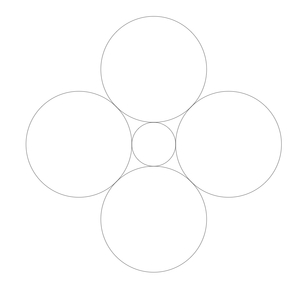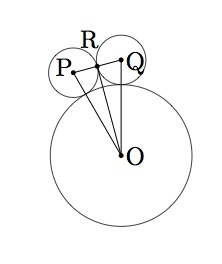# Seven Circles III

Alignments to Content Standards: G-SRT.C.8 G-SRT.D G-MG.A.1

Seven circles of the same size can be placed in the pattern shown below:The six outer circles touch the one in the center and each circle on the outside also touches its two neighbors in the outside ring.

1. If twelve circles are placed around a central circle, as pictured below, what is the relationship between the diameters of the outer circles and the diameter of the inner circle? Explain.2. If four circles are placed around a central circle, as pictured below, what is the relationship between the diameter of the outer circles and the diameter of the inner circle? Explain.## IM Commentary

This task is intended for instructional purposes only. It provides an opportunity to model a concrete situation with mathematics. Once a representative picture of the situation described in the problem is drawn (the teacher may provide guidance here as necessary), the solution of the task requires an understanding of the definition of the sine function. When the task is complete, new insight is shed on the ''Seven Circles I'' problem which initiated this investigation as is noted at the end of the solution.

This problem leads to what could make for a fun in class activity, namely measuring the diameters of different size coins (pennies, nickels, dimes, quarters, and half dollars) and using this information to estimate how many of each sized coin would fit around the circumference of a given coin. This information will be recorded in a separate task, ''Coins in a circular pattern.''

In the solution to both parts of this problem, the trigonometric function $\sin{x}$ needs to be evaluated, once for the benchmark angle of $45$ degrees and the other time for an angle of $15$ degrees. While not a benchmark angle, the value for $\sin(15)$ can be found using a double angle formula. In the second solution, the values for $\sin{x}$ are evaluated explicitly while in the first solution a calculator is used to find an approximate value.

## Solutions

Solution: 1

1. Let $O$ be the center of the central circle and $P$, $Q$ centers of adjacent circles in the ring and $R$ the midpoint of segment $PQ$ as pictured below:Let $r$ be the radius of the inner circle and $s$ the radii of the twelve outer circles. Segment $PO$ is congruent to segement $QO$ as both have a length of $s + r$. Segment $PR$ is congruent to segment $QR$ because $R$ is the midpoint of $PQ$. Finally segment $OR$ is congruent to segment $OR$. By $SSS$ we conclude that triangle $POR$ is congurent to triangle $QOR$. Since angles $ORP$ and $ORQ$ are congruent and, taken together, add up to $180$ degrees they are both right angles. Because there are twelve equal circles in the ring, the measure of angle $POQ$ is one twelfth of $360$ degrees or $30$ degrees. Angles $POR$ and $QOR$ are congruent and so they much each be $15$ degrees.

The sine of angle $POR$ is the length of the side opposite the angle, $|PR|$ divided by the length of the hypotenuse $|OP|$. So we have \begin{eqnarray} \sin(15^\circ) &=& \frac{|PR|}{|OP|} \\ &=& \frac{s}{r+s}. \end{eqnarray} Multiplying both sides by $r+s$ gives $r\sin{15^\circ} + s\sin{15^\circ} = s$. Solving for $s$ in terms of $r$ we find $$s = \left(\frac{ \sin{15^\circ}}{1-\sin{15^\circ}}\right) r.$$ For students who know double angle formulas, $\sin{15}$ can be calculated directly as in the second solution below. Evaluating on a calculator, we find that $s$ is about $0.349r$: as the picture shows, the circles in the ring are substantially smaller than the inner circle. For part (b) below, we should find, if the picture is accurate, that $s$ is substantially larger than $r$. The case where $s = r$ was seen in ''Seven Circles I.''
2. The method used in part (a) works more generally as we will verify here for the case where there are four circles in the ring. We continue to denote the radius of the inner circle by $r$ but use $t$ this time for the radius of the outer circle. Choosing $P$ and $Q$ to be centers of adjacent circles in the outer ring and $R$ the midpoint of segment $PQ$ we find the following picture:The remainder of the argument from part (a) now applies, the only change being that the measure of angle $POR$ is now $45$ degrees instead of $15$ degrees. The sine of angle $POR$ is the length of the side opposite the angle, $|PR|$ divided by the length of the hypotenuse $|OP|$. So we have \begin{eqnarray} \sin(45^\circ) &=& \frac{|PR|}{|OP|} \\ &=& \frac{t}{r+t}. \end{eqnarray} Multiplying both sides by $r+t$ gives $r\sin{45^\circ} +t\sin{45^\circ} = t$. Solving for $t$ in terms of $r$ we find $$t = \left(\frac{ \sin{45^\circ}}{1-\sin{45^\circ}}\right) r.$$ Here students may use the fact that $\sin{45} = \frac{\sqrt{2}}{2}$ as is done in the second solution below. Evaluating on a calculator, we find that $t$ is about $2.41r$, validating the picture which shows that the circles in the outer ring are substantially larger than the central circle.

Now that all of this work has been done we can check, as was asked in ''Seven Circles I'' that if six circles of radius $r^\prime$ are places around a circle of radius $r$ in the pattern shown at the beginning of the problem, then $$r^\prime = \left(\frac{ \sin{30^\circ}}{1-\sin{30^\circ}}\right) r = r$$ so that all seven circles in this pattern have the same size.

Solution: 2 Double angle formula

1. In order to calculate $\sin{15^\circ}$ exactly we may use the double angle formula for the cosine which is $$\cos{2x} = 1 - 2\sin^2{x}.$$ Plugging in $x = 15^\circ$ we find $$1 - 2\sin^2{15^\circ} = \cos{30^\circ} = \frac{\sqrt{3}}{2}.$$ Solving for $\sin{15^\circ}$ we find
\begin{align} \sin{15^\circ} &= \sqrt{\frac{1}{2} - \frac{\sqrt{3}}{4}} \\ &= \frac{\sqrt{2 - \sqrt{3}}}{2}. \end{align}
This exact expression can then be used to find the exact relationship between $s$ and $r$ since we know from the first solution that $$s = \left( \frac{\sin{15^\circ}}{1-\sin{15^\circ}}\right)r.$$ Simplifying this expression any further is rather tedious, but one ends with, among other possible expressions, the answer $$s = \left(\frac{\sqrt{3}-1}{1+2\sqrt{2}-\sqrt{3}}\right) r.$$
2. For part $b$ finding and exact value for $t$ is simpler than for part (a) because $\sin{45^\circ} = \frac{\sqrt{2}}{2}$. Using this we find
\begin{align} t &= \left( \frac{\sin{45^\circ}}{1-\sin{45^\circ}}\right)r \\ &= \left( \frac{\frac{\sqrt{2}}{2}}{1-\frac{\sqrt{2}}{2}}\right)r \\ &= (\sqrt{2} +1) r. \end{align}